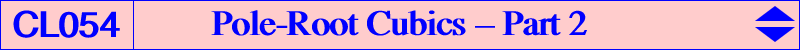Let us consider a non-pivotal cubic nK0(Ω,P) with pole Ω = (p:q:r) and root R = (u:v:w). nK0 is an equilateral cubic if and only if – either its root R is the transform of its pole Ω under the mapping ΩRe : Ω -> R, where R is the intersection of the line Ω-ΩRc(Ω) with the line passing through G and the Ω–isoconjugate of X(1989), – or its pole Ω is the transform of its root R under the mapping RΩe : R -> Ω, where Ω is a point on the line through RΩc(R) and the barycentric product of R and the infinite point of the line GR. These two mappings have the same fixed points namely A, B, C and K. Each mapping has three singular points : – for RΩe : G, L1, L2, these latter two points are the intersections of the circumconic with perspector X(50) with the trilinear polar of the barycentric quotient X(1989)÷X(381) i.e. the homologue of the midpoint of GH in the isoconjugation that swaps the Fermat points. – for ΩRe : X(1989), K1, K2, these latter two points are the infinite points of the circum-conic with perspector X(32) and their barycentric product is X(32). *** ΩRe maps any point on the line at infinity onto G and RΩe maps any point on the trilinear polar of X(1989)÷X(381) onto X(1989). In other words, – there are infinitely many equilateral nK0s with root G and pole on the line at infinity but these cubics always decompose into the line at infinity and the circumconic which is its isoconjugate, – and infinitely many equilateral nK0s with pole X(1989) and with root on the trilinear polar above. See K205 = nK0+(X1989, X523) and K501 = nK0(X1989, X1989 x X525) for example. From this, we see that ΩRe transforms a line (L) into a conic (C) passing through G, L1, L2 and RΩe transforms (L) into a conic (C') passing through X(1989), K1, K2. These two correspondences lead to two families of interesting cubics Ke1(Q) and Ke2(Q) when we look at the intersections of a line passing through a fixed point Q and (C) or (C'). This has to be compared with the definition of pivotal isocubics although the two mappings above are not involutions but simply reciprocal transformations. See also CL052. Note that, for a given point Q, the pencil generated by Ke1(Q) and Ke2(Q) contains the pivotal cubic pK(gtQ, K) where gtQ is the isogonal of the isotomic of Q. This is the pK with pivot K and isopivot Q. *** The cubics Ke1(Q) These are the cubics Ke1(Q) obtained when we intersect the line (L) and the conic (C). For a given point Q, the cubic Ke1(Q) is a circum-cubic that also contains G, K, L1, L2, Q, ΩRe(Q). Its meets the sidelines of ABC at U, V, W. This family of cubics contains : – K0s if and only if Q lies on the Euler line and, in this case, it contains X(378). See K280 = Ke1(X2). – cubics such that ABC and UVW are perspective when Q lies on a circumcubic through X(3), X(4), X(5), X(64). This gives three pKs namely Ke1(X3), Ke1(X4) and K502 = Ke1(X5) which is an isogonal pivotal cubic. – cubics such that U, V, W are collinear when Q lies on a circumcubic through X(110), X(1989) giving two decomposed cubics, X(523) giving K397 and X(1495) giving K503, a nK isocubic. – one circular cubic for an unknown point Q on the Euler line, – one equilateral cubic for a rather complicated Q, – K+ cubics for Q on a non-cicumcubic without any known center.*** The cubics Ke2(Q) These are the cubics Ke2(Q) obtained when we intersect the line (L) and the conic (C'). For a given point Q, the cubic Ke2(Q) is a circum-cubic that also contains K, X(1989), K1, K2, Q, RΩe(Q). These are precisely the cubics Kc2(Q) we met in CL053.